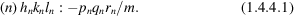International
Tables for
Crystallography
Volume B
Reciprocal space
Edited by U. Shmueli

International Tables for Crystallography (2010). Vol. B, ch. 1.4, pp. 119-120   | 1 | 2 |

## Section 1.4.4.2. Arrangement of the space-group tables

U. Shmuelia

#### 1.4.4.2. Arrangement of the space-group tables

| top | pdf |

Table A1.4.4.1is subdivided into point-group sections and space-group subsections, as outlined below.

 (i) The point-group heading. This heading contains a short Hermann–Mauguin symbol of a point group, the crystal system and the symbol of the Laue group with which the point group is associated. Each point-group heading is followed by the set of space groups which are isomorphic to the point group indicated, the set being enclosed within a box. (ii) The space-group heading. This heading contains, for each space group listed in Volume A (IT A, 1983), the short Hermann–Mauguin symbol of the space group, its conventional space-group number and (in parentheses) the serial number of its representation in Volume A; this is also the serial number of the explicit space-group symbol in Table A1.4.2.1 from which the entry was derived. Additional items are full space-group symbols, given only for the monoclinic space groups in their settings that are given in Volume A (IT, 1983), and self-explanatory comments as required. (iii) The table entry. In the context of the analysis in Section 1.4.2.2, the format of a table entry is:, whereis the nth space-group operator, and the phase shiftis expressed in units of 2π [see equations (1.4.2.3)and (1.4.2.5)]. More explicitly, the general format of a table entry isIn (1.4.4.1), n is the serial number of the space-group operation to which this entry pertains and is the same as the number of the general Wyckoff position generated by this operation and given in IT A (1983)for the space group appearing in the space-group heading. The first part of an entry,:, contains the coordinates of the reciprocal-lattice vector that was generated from the reference vector (hkl) by the rotation part of the nth space-group operation. These rotation parts of the table entries, for a given space group, thus constitute the set of reciprocal-lattice points that are generated by the corresponding point group (not Laue group). The second part of an entry is an abbreviation of the phase shift which is associated with the nth operation and thuswhere the fractionsandare the components of the translation partof the nth space-group operation. The phase-shift part of an entry is given only ifis not a vector in the direct lattice, since such a vector would give rise to a trivial phase shift (an integer multiple of 2π).

### References

International Tables for Crystallography (1983). Vol. A, Space-Group Symmetry, edited by Th. Hahn. Dordrecht: Reidel.